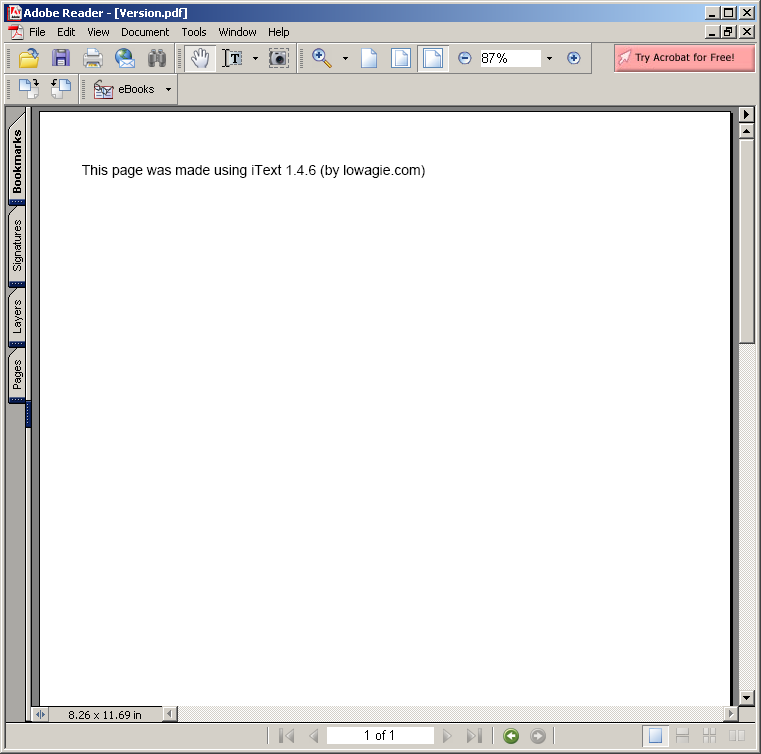# Algorithms « Collections Data Structure « Java

1.Anagrams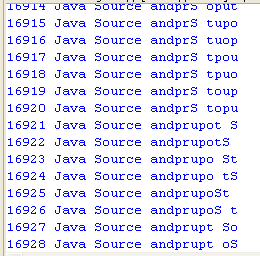2.Hanoi puzzle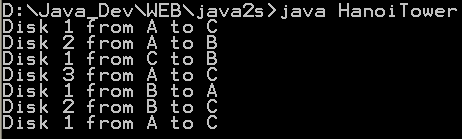3.Fibonacci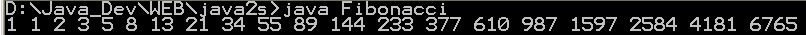4.Sieve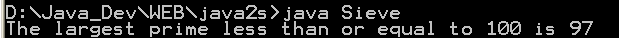5.Find connections using a depth-first search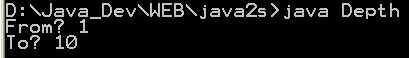6.Find connections using hill climbing.
7.Find optimal solution using least-cost
8.Find the lost keys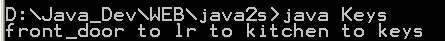9.Compute the area of a triangle using Heron's Formula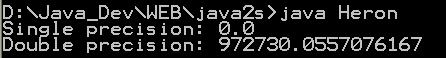10.Compute prime numbers
11.Print a table of fahrenheit and celsius temperatures 1
12.Print a table of fahrenheit and celsius temperatures 2
13.Print a table of Fahrenheit and Celsius temperatures 3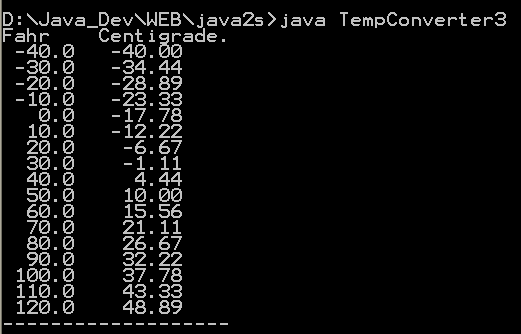14.Soundex - the Soundex Algorithm, as described by Knuth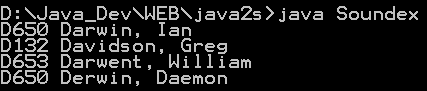15.A programmable Finite State Machine implementation.
16.An extendable Graph datastructure.
17.Utilities for flop (floating-point operation) counting.
18.LU Decomposition
19.Reverse Polish Notation
20.Permutator test
21.implements the LZF lossless data compression algorithm
22.Linear Interpolation
23.Utility class for generating the k-subsets of the numbers 0 to n
24.Version